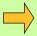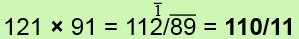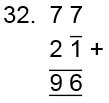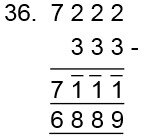Wallis wrote in a letter in 1669: "In a dark night in bed, without pen, ink or paper or anything equivalent, I did by memory extract the square root of 3,00000, 00000, 00000, 00000, 00000, 00000, 00000, 00000 which I found to be 1,73205, 08075, 68077, 29353, feré, and did the next day commit to writing."

"February 18th 1670, Joannes Georgius Pelshower giving me a visit, and desiring an example of the like, I did that night propose to myself in the dark without help to my memory a number in 53 places: 24681, 35791, 01112, 14111, 31516, 18201, 71921, 22242, 62830, 23252, 72931 of which I extracted the square root in 27 places: 15,71030, 16871, 48280, 58171, 52171 proxima."

John Wallis (1616-1703) was a brilliant mathematician and a contemporary of Isaac Newton. In addition to his works on pure mathematics he also wrote on astronomy, the tides, the laws of motion, botany, physiology, music, geology etc.

Chapter 5

All From 9 and the Last From 10

This chapter shows a surprisingly easy way of multiplying numbers near a base, near different bases, or near multiples of a base, and has a considerable range. The use of negative numbers which can enormously simplify calculations is introduced, and applications in addition and subtraction are also included.

Numbers Just Below a Base

In the conventional system of mathematics a sum like 88 × 98 is considered especially difficult because of the large figures, 8 and 9.

But since the numbers 88 and 98 are close to the base of 100 we may think that there ought to be an easy way to find such a product.

In the Vedic system this kind of sum is extremely easy however. We simply note that both numbers are close to 100, and that 88 is 12 below 100, and 98 is 2 below 100, and we just give the answer:Example 1The deficiencies (12 and 2) have been written above the numbers (on the flag), the minus signs indicating that the numbers are below 100.

The answer 8624 is in two parts: 86 and 24.
The 86 is found by taking one of the deficiencies from the other number.
That is:

88 - 2 = 86 or 98 - 12 = 86 (whichever you like),

and the 24 is simply the product of the deficiencies: 12 × 2 = 24.
It could hardly be easier.Example 2

SimilarlyThe differences from 100 are 7 and 4,
93 - 4 = 89 or 96 - 7 = 89,
and 7 × 4 = 28.Example 3

AlsoNote the zero inserted here: the numbers being multiplied are near to 100, so two digits are required on the right, as in the other examples.

Question Comment !
 Tutorial Title Question Title No Questions 0 Questions Asked 0 Skipped Questions 0 Correctly Answered 0 Wrongly Answered 0 Total Question Attempts 0 Current Question Attempts 0

Skip Question

Reset Test

Halt Test

 No Questions 0 Questions Asked 0 Skipped Questions 0 Correctly Answered 0 Wrongly Answered 0 Total Question Attempts 0

Time Taken

CloseExercise AExample 4Here the numbers are each 11 below 100, and 11 × 11 = 121, a 3-figure number. The hundreds digit of this is therefore carried over to the left.

So the most efficient mental procedure is to take one number and subtract the other deficiency from it. Then multiply the deficiencies together, mentally adjusting the first part of the answer if there is a carry figure.Exercise B

Find 92 × 196 by

a) thinking of 196 as1/96,
b) using proportionally and All from 9 and the Last from 10,
c) using proportionally and By One More than the One BeforeExample 5

568 × 998 = 566864

We see here that the numbers 568 and 998 are conveniently close to 1000, so we allow 3 figures on the right. The differences of the numbers from 1000 are 432 and 2.

The method is just the same:
568 - 2 = 566,
432 × 2 = 864.

However in the case of 568 here the deficiency is not so obvious as in the previous examples, and this is exactly where the Sutra of the present chapter comes in.

If All From 9 and the Last From 10 is applied to the digits of 568 we get 432:

take the 5 from 9 and get 4,
take the 6 from 9 and get 3,
we take the 8 from 10 and get 2.

This formula gives the deficiency of any number from the next highest base. It could have been applied in the previous examples too: for 88, in the first example, we take the first 8 from 9 to get 1, and the last from 10 to get 2. This gives the deficiency of 12 below the base of 100. For 98 we get 02, or just 2.

Subtraction

Thus All From 9 and the Last From 10 provides us also with a very effective method of subtraction from a base number:

1000 - 587 = 413,

10000 - 785 = 10000 - 0785 = 9215, and so on.

Also 7000 - 111 = 6889, the 7 is reduced to a 6 because 111 is to be taken from one of the 7 thousands, so only 6 thousands are left, and the Sutra is applied to 111 to get 889.

Thus frequent subtraction problems involving money etc. are quickly solved by this method:

for example £70.00 - £1.11 = £68.89.

We may also write 7000 - 111 = 7111 in which we put a bar (called a "vinculum") over the 111 to show that it is negative.

This subtraction method is completely general, covering all types of subtraction:

7654 - 1928 = 6334 (since 7-1= 6, 6-9 = 3, 5-2 = 3, 4-8 = 4)

and 6334 = 63/34 (since 60-3 = 57, 30-4 = 26).

We will see subtractions like this coming up in some of the later multiplication devices.Exercise CExample 6

58776 × 99998 = 58774/82448

58776 - 2 = 58774, and the formula gives the deficiencies 41224, 2 which are multiplied together to get 82448.Example 7

Similarly 857 × 994 = 851858

where 851 = 857 - 6, and 858 = 143 × 6.Example 8

7 × 8 = 56

Multiplication tables above 5 × 5 are not essential in the Vedic system.

Here the deficiencies are 3 and 2 as we take 7 and 8 from 10 (...Last from 10):
7 - 2 = 5 and 3 × 2 = 6, therefore 7 × 8 = 56.Exercise D

Division by numbers near a base (above or below the base) includes the use of the Sutra Vertically and Crosswise as well as the Sutra of the present chapter. It is therefore shown at the end of the next chapter.

We now extend this simple multiplication technique in several different directions.

Numbers Above A Base

First let us suppose that the numbers being multiplied are both above a base, rather than below it.Example 9

103 × 104 = 10712

This is even easier than the previous examples, but the method is just the same. The deficiencies are +3 and +4: positive now because the numbers are above the base.

103 + 4 = 107 or 104 + 3 = 107, and 4 × 3 = 12.Example 10

12 × 13 = 156 (12+3=15, 2×3=6)Example 11

1234 × 1003 = 1237702 (1234+3=1237, 234×3=702)Example 12

10021 × 10002 = 100230042 (10021+2=10023, 0021×2=0042)Exercise E

One Number Above and One Number Below the BaseExample 13

124 × 98 = 12248 = 12152

Here the base is 100 and the deficiencies from 100 are +24 and -2.

Applying the usual procedure we find 124 - 2 = 122 or 98 + 24 = 122.
So 122 is the left-hand part of the answer.
Then multiplying the deficiencies we get -48, written 48 (since a plus times a minus gives a minus). This gives the answer as 12248.
To remove the negative portion of the answer we just take 48 from one of the hundreds in the hundreds column. This simply means reducing the hundreds column by 1 and applying All From 9 and the Last From 10 to 48. Thus 122 becomes 121 and 48 becomes 52.
So 12248 = 12152.Example 14

1003 × 987 = 990/039 = 989/961

Similarly, we first get 1003-13 = 990 or 987+3 = 990,
and +3 × -13 = 039 (3 figures required here as the base is 1000).
Then 990 is reduced by 1 to 989, and applying the formula to 039 gives 961.Example 15Here we have a minus one to carry over to the left so that the 112 is reduced by 2 altogether.Exercise F

Proportionally

Here we bring in the formula from Chapter 2.Example 16

213 × 203 = 2 × 216/39 = 43239

We observe here that the numbers are not near any of bases used before: 10, 100, 1000 etc. But they are close to 200, with deficiencies of 13 and 3.
The usual procedure gives us 216/39 (213+3=216, 13×3=39).
Now since our base is 200 which is 100×2 we multiply only the left-hand part of the answer by 2 to get 43239.Example 17

29 × 28 = 3×27/2 = 812

The base is 30 (3×10), and the deficiencies are -1 and -2.
29-2 = 27, 1×2 = 2 and 3×27 = 81.Example 18

311 × 298 = 3×309/22 = 926/78

Here the numbers are above and below 300: we multiply the left-hand side by 3 before deducting 1 to deal with the negative right-hand portion.

Thus the Proportionately formula extends considerably the range of the method.
The only additional step being the multiplication of the left-hand part of the answer. One further application of this formula may also be noted:Example 19

88 × 49 = half of (88×98) = half of (8624) = 4312Exercise G

Numbers Near Different BasesExample 20

9998 × 94 = 9398/12

The bases here are 10,000 and 100 and the deficiencies are -2 and -6.
The answer is in two parts: 9398 and 12.
In subtracting the deficiency 6 from the first number, imagine the numbers are under each other:

9998
94

and subtract the 6 in the column indicated by the last figure of the smaller number, that is, the second column from the left here.
So 9998 becomes 9398.
Then multiply the deficiencies together: 2×6 = 12.

Note that the number of figures in the right-hand part of the answer corresponds to the base of the lower number (94 is near 100, therefore there are 2 figures on the right).Example 21

10007 × 1003 = 10037021

Lining the numbers up:we see that the deficiency 3 is added in the 4th column, giving 10037.

The product of the deficiencies is 7×3=21, but as the base of the smaller number is 1000 we need 3 figures on the right, so we put 021.Example 22

1032 × 98 = 1012/64 = 101136

Note here that because 98 = 102 the deficiency 2 is deducted from the 3 to give 1012 on the left.Exercise H

Multiplying Three Numbers SimultaneouslyExample 23

98 × 97 × 96 = 91/26/24 = 912576

These numbers are all close to 100, their deficiencies being 2, 3, 4.
The answer is in 3 parts as indicated by the oblique lines.
First take one number and take both of the other deficiencies from it.
For example 98-3-4 = 91. This is the first part of the answer.

Then multiply the deficiencies together in pairs and add the results up:
2×3 + 2×4 + 3×4 = 6+8+12 = 26.
This is the middle part of the answer.

Finally just multiply all 3 deficiencies together: 2 × 3 × 4 = 24.
We write 24 because the deficiencies are actually negative.
The 24 is then removed as in Examples 13 and 14 earlier.Example 24

1022 × 1002 × 1003 = 1027/116/132

1022+2+3 = 1027, 22×2 + 22×3 + 2×3 = 116, 22×2×3 = 132.Exercise I

Squaring Numbers Near A Base

This is an especially easy case under the present formula, which is described by the sub-formula Reduce (or increase) by the Deficiency and also set up the square.Example 25

962 = 92/16

96 is 4 below 100, so we reduce 96 by 4, which gives us the first part of the answer. The last part is just 42 = 16 as the formula says.Example 26

10062 = 1012/036

Here 1006 is increased by 6 to 1012, and 62 = 36: but with a base of 1000 we need 3 figures on the right, so we put 036.Example 27

3042 = 3×308/16 = 92416

This is the same but because our base is 300 the left-hand part of the answer is multiplied by 3.

Note the following alternative method: if we look at the number split so that
3042 = 9/24/16, then we may see that 9 = 32,
24 = twice 3 × 4, and 16 = 42.Exercise J

Squaring Numbers Near 50

It is worth noting this case, which also comes under the above formula.Example 28

542 = 29/16

Since 502 = 2500 and we have 542, which is 4 more,
we have 25 + 4 = 29,
and 42 = 16.Example 29

482 = 2304

25 - 2 = 23 (25, as before, minus the deficiency, 2)
22 = 4 (the deficiency squared)Exercise K

Multiplication By Nines

The Vedic formula By One Less Than the One Before, which is the converse of the formula of the previous chapter, comes in here in combination with All From 9 and the Last From 10.Example 30

763 × 999 = 762/237

The number being multiplied by 9's is first reduced by 1:763-1 = 762, then All From 9 and the Last From 10 is applied to 763 to get 237.Example 31

1867 × 99999 = 1866/98133

Here, as 1867 has 4 figures, and 99999 has 5 figures, we suppose 1867 to be 01867. This is reduced by 1 to give 1866,
and applying All From 9.... to 01867 gives 98133.Exercise LExample 32

77 + 19 = 96

If asked to add 19 to a number we would probably add 20 and take one away, because 19 = 20-1.Example 33

365 + 177 = 542

In adding 177 we might add 200 and subtract 23.
Note that we get 23 by applying All From 9... to 77.Exercise M

SubtractionExample 34

77 - 19 = 58

Similarly here, the natural thing to do is to subtract 20 and add 1 back on.Example 35

5040 - 1688 = 3352

We subtract 2000 and add 312: the All From 9... value of 688.Example 36

7222 - 333 = 7000 - 111 = 6889

Here the natural thing to do is probably to observe that we can easily subtract 222 of the 333, leaving 111 to be subtracted from 7000.
This last step also involves All From 9... as discussed at the end of Example 5.Exercise N

Writing Addition and Subtraction Sums

Earlier in this chapter we made use of negative numbers. We have written for -48, for example. This bar on the top of a number is called a vinculum. The use of this vinculum enormously simplifies many calculations. Below we give, without explanations , the equivalent written forms of examples 32 to 36.